# Initial Hypothesis

In a recent blog post, I used Tableau to create a visualization of total taxes collected versus population for municipalities in British Columbia (BC) and Alberta (AB). This plot has been recreated below in R. Note that both axes are logged in order to make the graph more readable:

``````#Replication of Tableau visualization
#Assume library(tidyverse) has been run and data loaded into a tibble called "dat"
ggplot(dat, mapping=aes(x=log10(Population), y=log10(TotalTax), color=JurisCode)) +
geom_point(shape = 1, size=3) +
scale_shape(solid=FALSE) +
ylab("Total Tax (log)") +
xlab("Population (log)") +
geom_smooth(method=lm, se=FALSE)``````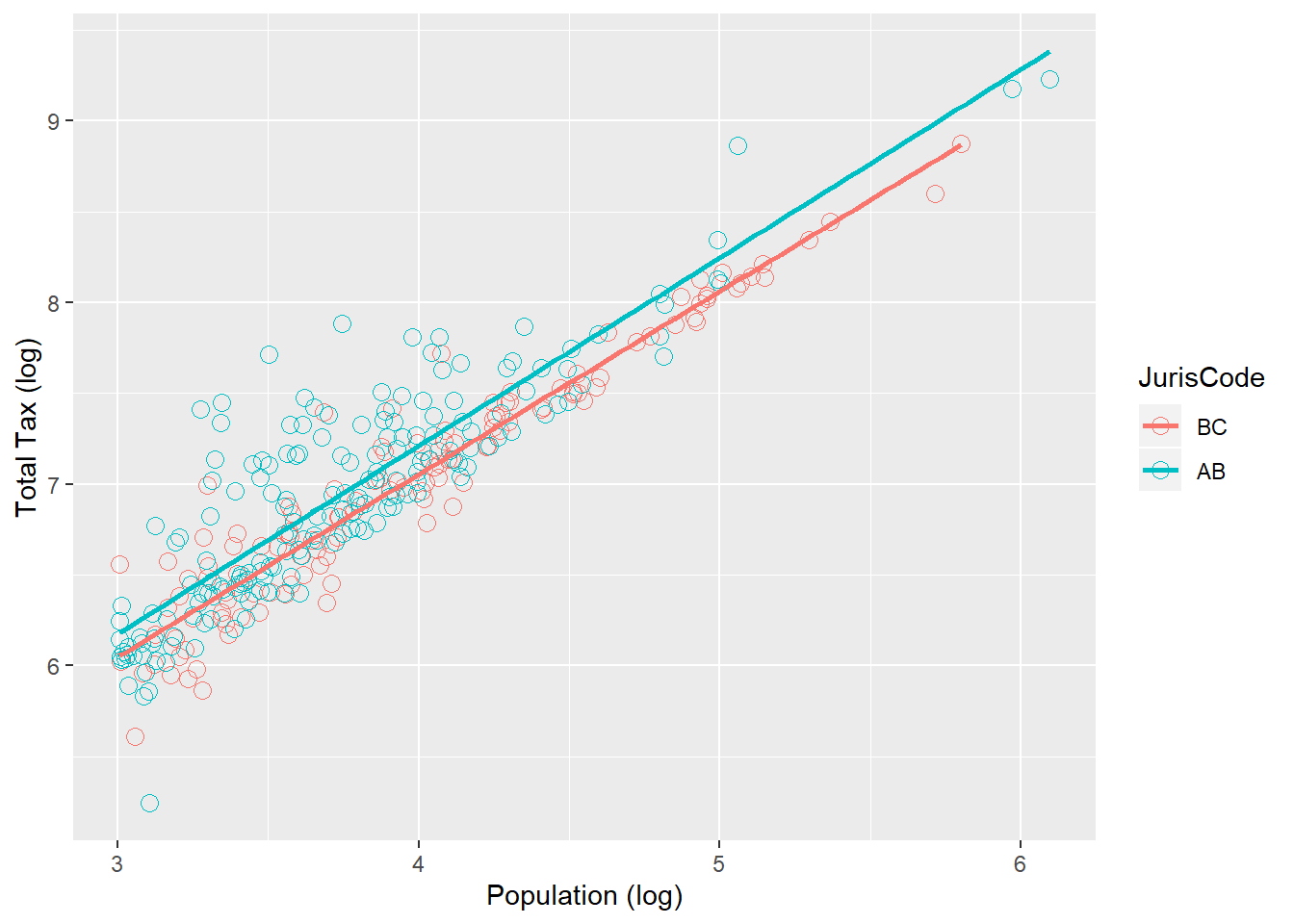The best-fit lines suggests that, on average, AB municipalities have higher rates of per capita taxes than BC municipalities. This is certainly an interesting result. But, as is always the case, a two-dimensional visualization is a good place to generate hypotheses. But to test hypotheses, we need to use statistical tools.

# Analysis

In the interest of repicability, I include the full R code here. Readers with no interest in R can skip the code and focus on the results. The data is available by request.

## Preliminaries

I start by loading the necessary libraries and performing some basic filtering and recoding of the data. Specifically, I censor all municipalities with population less than 1,000 and zero taxes (missing data). Then, I log both population and taxes to recreate the relatively clean linear relationship shown in the visualization. Finally, I transform the BC/AB field (`JurisCode`) into a factor variable. This simplifies subsequent analysis. Finally, I use the `summary` function to provide descriptive statistics for all the variables in the dataframe.

``````#Preliminaries (libraries and data preparation)
library(tidyverse)
library(QuantPsyc)
library(broom)
dat <- dat %>% filter(TotalTax > 0 & Population > 1000)
dat <- dat %>% mutate(JurisCode = as_factor(JurisCode),
PerCapitaTax = TotalTax/Population,
logTotalTax = log(TotalTax),
logPopulation = log(Population),
logTaxableLand = log(TaxableLand))

summary(dat)``````
``````##      OrgID            YearID     OrgNameFull          OrgName
##  Min.   :  1.00   Min.   :2017   Length:310         Length:310
##  1st Qu.: 82.25   1st Qu.:2017   Class :character   Class :character
##  Median :241.50   Median :2017   Mode  :character   Mode  :character
##  Mean   :249.67   Mean   :2017
##  3rd Qu.:402.75   3rd Qu.:2017
##  Max.   :536.00   Max.   :2017
##     Latitude       Longitude      JurisCode   Population
##  Min.   :48.37   Min.   :-130.3   BC:128    Min.   :   1015
##  1st Qu.:49.59   1st Qu.:-121.7   AB:182    1st Qu.:   2442
##  Median :51.43   Median :-115.8             Median :   5376
##  Mean   :51.79   Mean   :-117.2             Mean   :  26313
##  3rd Qu.:53.71   3rd Qu.:-113.4             3rd Qu.:  13070
##  Max.   :59.09   Max.   :-110.0             Max.   :1246337
##   TaxableLand         TotalTax          PerCapitaTax      logTotalTax
##  Min.   :      3   Min.   :1.742e+05   Min.   :  136.3   Min.   :12.07
##  1st Qu.:    812   1st Qu.:2.915e+06   1st Qu.:  956.8   1st Qu.:14.89
##  Median :   2584   Median :8.940e+06   Median : 1182.1   Median :16.01
##  Mean   : 216327   Mean   :3.772e+07   Mean   : 1782.5   Mean   :16.05
##  3rd Qu.:  21845   3rd Qu.:2.386e+07   3rd Qu.: 1692.2   3rd Qu.:16.99
##  Max.   :8458900   Max.   :1.702e+09   Max.   :16317.3   Max.   :21.26
##  logPopulation    logTaxableLand
##  Min.   : 6.923   Min.   : 1.099
##  1st Qu.: 7.800   1st Qu.: 6.699
##  Median : 8.590   Median : 7.857
##  Mean   : 8.818   Mean   : 8.664
##  3rd Qu.: 9.478   3rd Qu.: 9.992
##  Max.   :14.036   Max.   :15.951``````

## Taxes versus Population

One nice thing about Tableau is that it fits a line to the data in a scatterplot and provides much of information from the underlying linear model. One bad thing about Tableau it is does not provide all of the information from the underlying linear model. Specifically, it does not provide regression diagnostics. Fitting a line to some data points is one thing; satisfying the assumptions of the underlying technique is another.

### Basic Tableau Model

Although I logged the axes in the Tableau visualization, Tableau creates its regression using the unlogged data. In addition, by default Tableau forces the intercept through the point (0,0). Although this makes for a more attractive graph, the practice of forcing the intercept is controversial (especially in this case, in which the intercept is well outside the sample—there are no functioning local governments with zero population).

Running separate zero-intercept regressions for BC and AB, we get roughly the same parameter estimates as Tableau. I am not exactly sure why they are not indentical, but it is hard to know what is going on under the hood in a tool like Tableau.

``````#Simple models for BC and AB
mod.simple.BC <- lm(TotalTax ~ 0 + Population, data=dat[dat\$JurisCode=='BC',])
summary(mod.simple.BC)``````
``````##
## Call:
## lm(formula = TotalTax ~ 0 + Population, data = dat[dat\$JurisCode ==
##     "BC", ])
##
## Residuals:
##        Min         1Q     Median         3Q        Max
## -152334878    -926128     556656    3293575   85501420
##
## Coefficients:
##            Estimate Std. Error t value Pr(>|t|)
## Population  1057.27      18.52    57.1   <2e-16 ***
## ---
## Signif. codes:  0 '***' 0.001 '**' 0.01 '*' 0.05 '.' 0.1 ' ' 1
##
## Residual standard error: 17910000 on 127 degrees of freedom
## Multiple R-squared:  0.9625, Adjusted R-squared:  0.9622
## F-statistic:  3260 on 1 and 127 DF,  p-value: < 2.2e-16``````
``````mod.simple.AB <- lm(TotalTax ~  0 + Population, data=dat[dat\$JurisCode=='AB',])
summary(mod.simple.AB)``````
``````##
## Call:
## lm(formula = TotalTax ~ 0 + Population, data = dat[dat\$JurisCode ==
##     "AB", ])
##
## Residuals:
##        Min         1Q     Median         3Q        Max
## -145801527   -1943548    -538694    5500207  558924406
##
## Coefficients:
##            Estimate Std. Error t value Pr(>|t|)
## Population  1482.51      29.09   50.96   <2e-16 ***
## ---
## Signif. codes:  0 '***' 0.001 '**' 0.01 '*' 0.05 '.' 0.1 ' ' 1
##
## Residual standard error: 45990000 on 181 degrees of freedom
## Multiple R-squared:  0.9348, Adjusted R-squared:  0.9345
## F-statistic:  2597 on 1 and 181 DF,  p-value: < 2.2e-16``````

The problem with these two parallel models is that, although they look good when plotted and have very high values for explained variance (\(R^2\)), the regression diagnostics suggest serious problems. Linear regression is finicky and its assumptions must be satisfied for its results to be legitimate. For example, a core requirement of regression is that residuals (difference between observed and predicted values) be “random” rather than attributable to a specific cause. In practical terms, this means the residuals should be normally distributed around a mean of zero. The plots of the residuals—which are not provided by Tableau’s simple line-fitting functionality—suggest some serious problems. The histogram of residuals for BC makes it clear that the errors are not random and the boxplot shows asymmetry in the form of many very large positive residuals. Thus, as is often the case, the complexities of real-world data compel us to go beyond the simple line-fitting functionality of Tableau or Excel and construct a more complex regression model.

``hist(resid(mod.simple.BC))``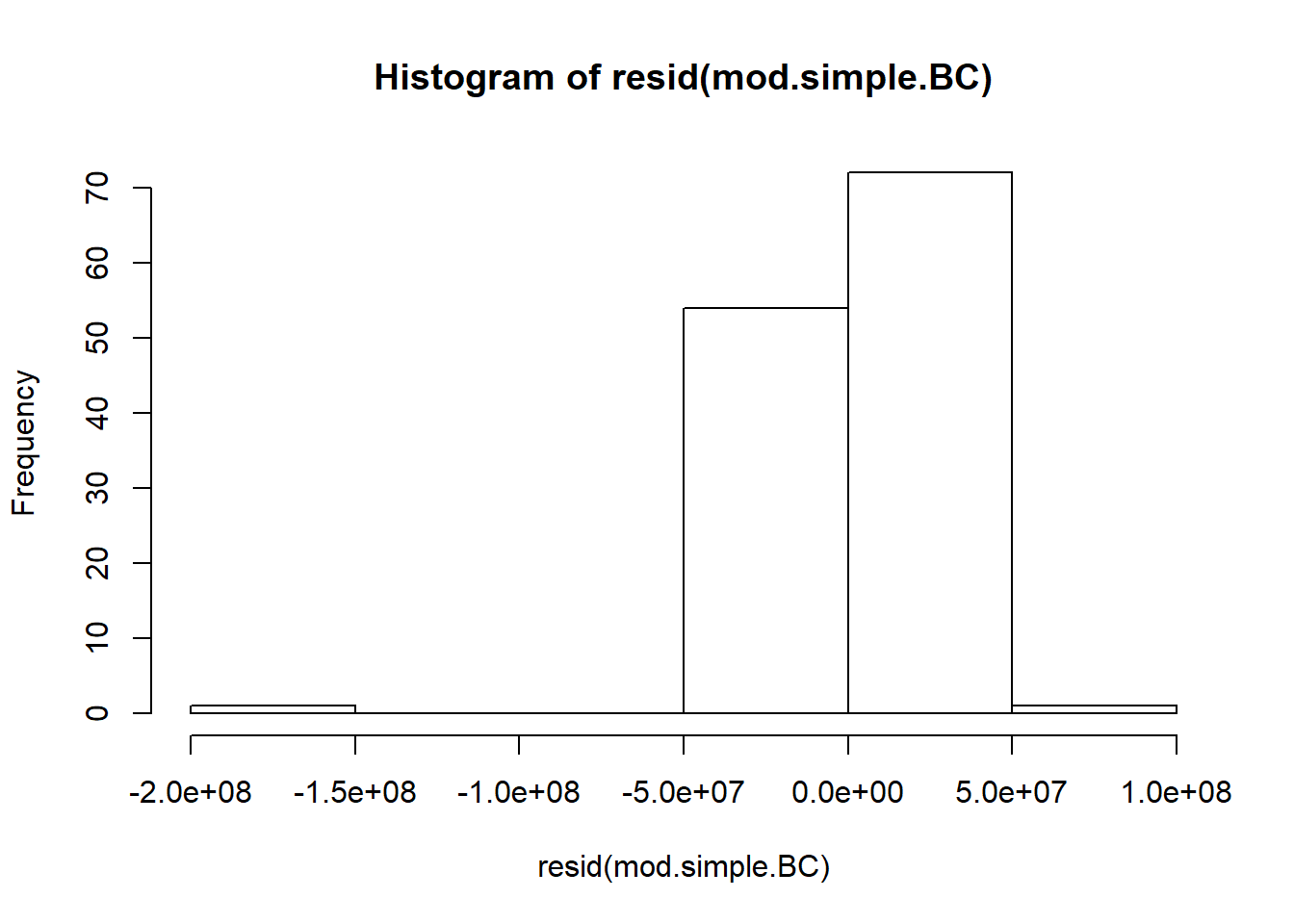``boxplot(resid(mod.simple.BC), horizontal = TRUE)``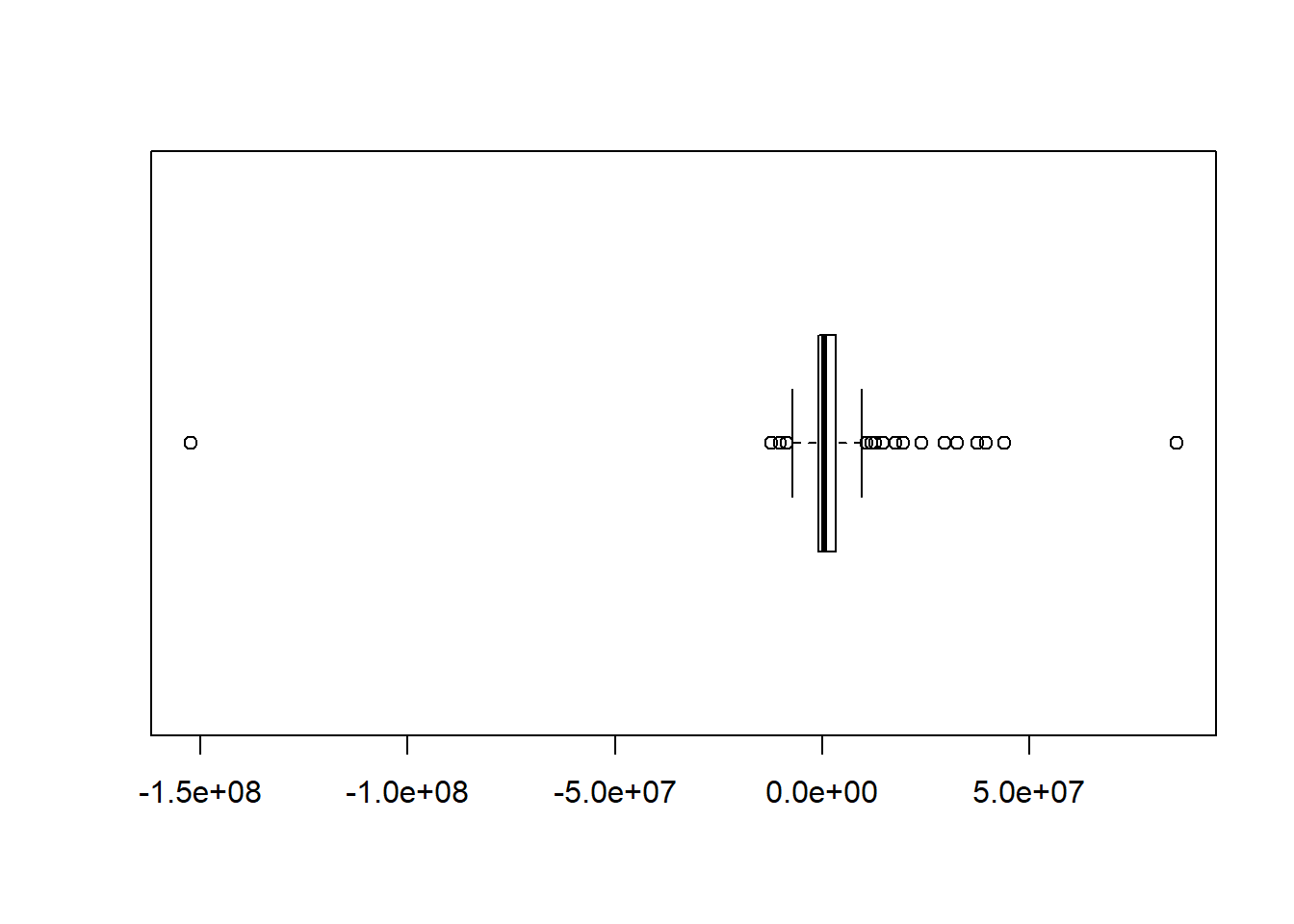### Improved Tableau model: logging population and taxes

Recall that the clean linear relationships in the visualization were the result of logging both axes. Indeed, if we unlog the axes in the visualization, we get a much less compelling representation of the relationship between taxation and population:

``````#Unlogged version of the Tableau visualization
ggplot(dat, mapping=aes(x=Population, y=TotalTax, color=JurisCode)) +
geom_point(shape = 1, size=3) +
scale_shape(solid=FALSE) +
ylab("Total Tax") +
xlab("Population") +
geom_smooth(method=lm, se=FALSE)``````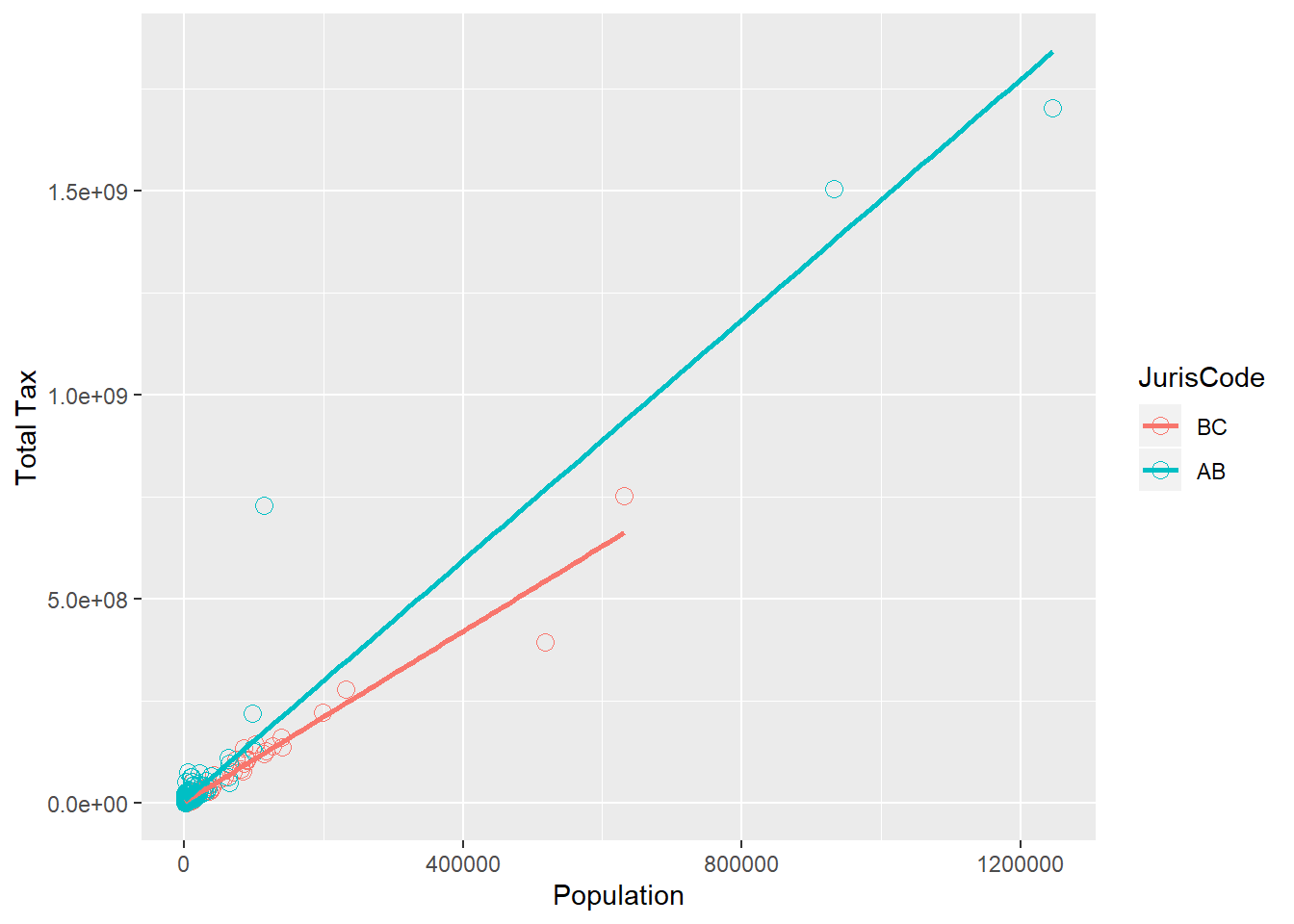Thus, an obvious first step to improving the regression model is to log taxes and population. Here I use the logged version of the variables created in the preliminaries:

``````mod.simple <- lm(logTotalTax ~ logPopulation + JurisCode, data=dat)
summary(mod.simple)``````
``````##
## Call:
## lm(formula = logTotalTax ~ logPopulation + JurisCode, data = dat)
##
## Residuals:
##     Min      1Q  Median      3Q     Max
## -2.4220 -0.3777 -0.1160  0.2694  2.3429
##
## Coefficients:
##               Estimate Std. Error t value Pr(>|t|)
## (Intercept)    6.83047    0.24557  27.815  < 2e-16 ***
## logPopulation  1.02190    0.02621  38.984  < 2e-16 ***
## JurisCodeAB    0.35001    0.07170   4.881  1.7e-06 ***
## ---
## Signif. codes:  0 '***' 0.001 '**' 0.01 '*' 0.05 '.' 0.1 ' ' 1
##
## Residual standard error: 0.6089 on 307 degrees of freedom
## Multiple R-squared:  0.8328, Adjusted R-squared:  0.8317
## F-statistic: 764.4 on 2 and 307 DF,  p-value: < 2.2e-16``````

Before we interpret this model, it is good practice to examine the residuals for normality. Below I show a histogram, boxplot, and a q-q plot. Although none of these suggests perfect normality, this is a huge improvement over the non-logged models. I therefore conclude that the model is good enough for high-level root cause analysis and move on to interpretation.

``hist(resid(mod.simple))``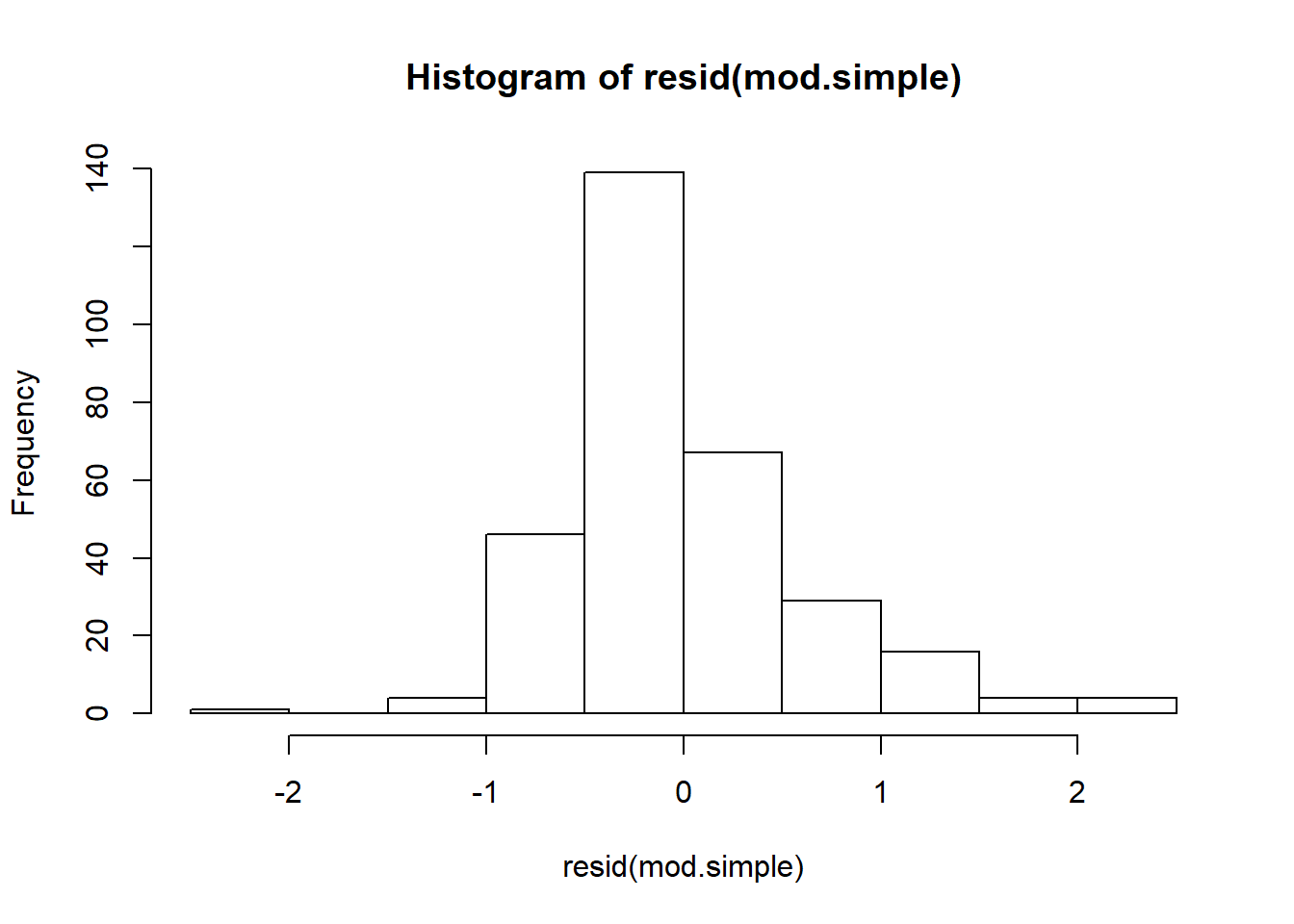``boxplot(resid(mod.simple), horizontal = TRUE)``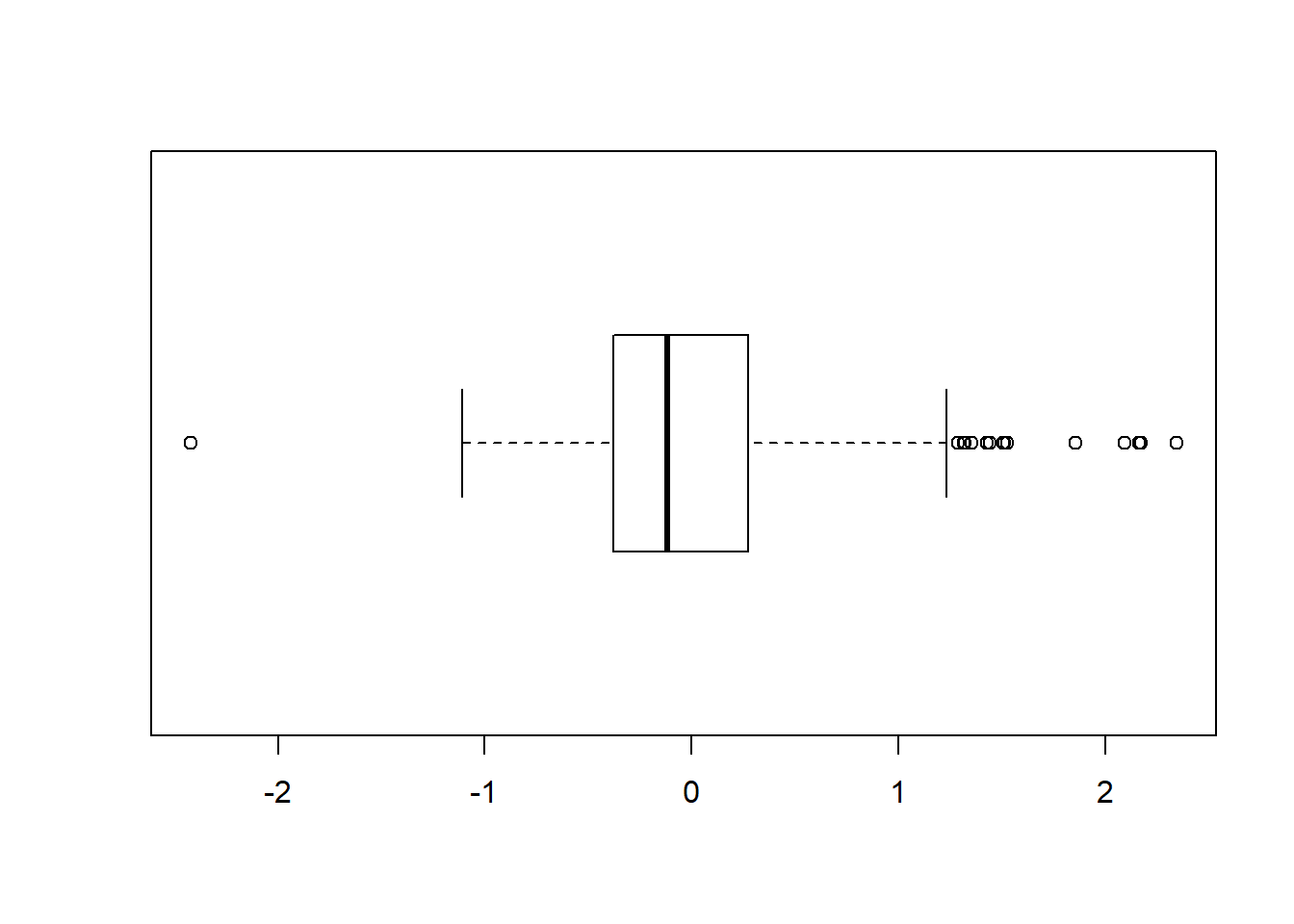``````qqnorm(resid(mod.simple))
qqline(resid(mod.simple))``````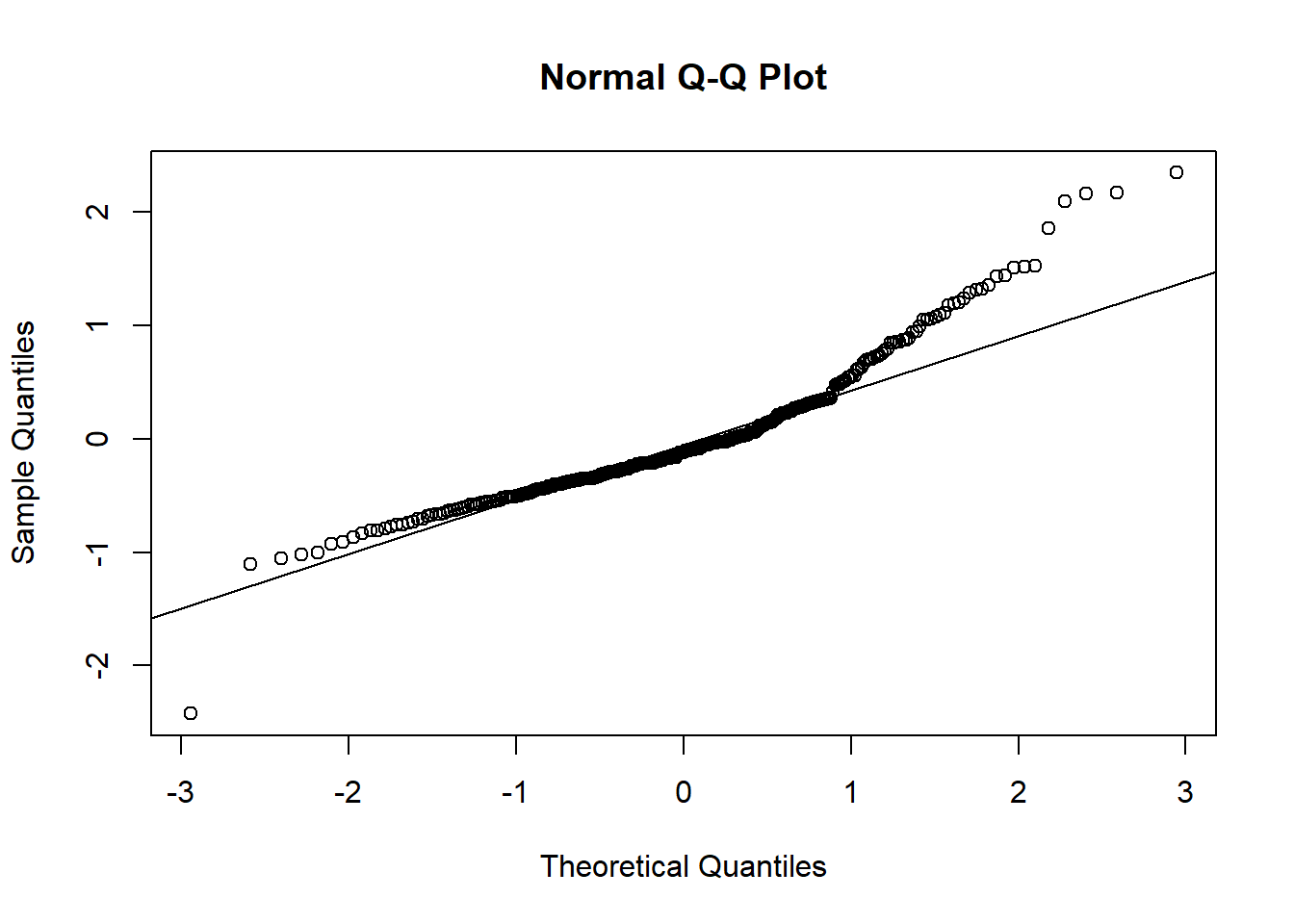The downside of logging one or more variables in regression model is that we lose the clean linear interpretation of the coefficient for each \(x_i\) as a slope. Reading the Tableau trendlines, we could say that each additional person in BC results in an average increase in taxation of \$1,058 and each additional person in AB results in an average increase in taxation of \$1,482. We know from the distribution of the residuals that these estimates are completely unreliable, but at least they are easy to understand. A more reliable conclusion is provided by the transformed model: the natural logarithm of taxes increases by 1.0219 when the natural logarithm of population increases by 1.0. Or, expressed as an equation \({taxes} = {population}^{1.0219}\). Not pretty or intuitive, but our world is not guaranteed to be linear.

A more practical interpretation of the transformed model simply focuses on the significance of the coefficients:

``````logPopulation  1.02190    0.02621  38.984  < 2e-16 ***
JurisCodeAB    0.35001    0.07170   4.881  1.7e-06 ***``````

First, we note that the coefficient of `logPopulation` is highly significant. This comes as no surprise: the whole take-away from the Tableau visualization is that population almost completely determine taxes. Second, the coefficient for the dummy variable `JurisCodeAB` is positive and highly significant. What this suggests (keeping in mind that our response variable is natural logged) is that, controlling for population, Albertans pay 35% more tax than British Columbians. This is an interesting result given is thorny political implications. But before we ascribe differences in taxation to political jurisdiction, we need to ask whether there are other, more obvious, explanations.

## A More Complex Model

When we build explanatory models, we invariably run into practical limitations. First, we can only test hypotheses that we can imagine. Thus, we have to know something about the underlying mechanisms of the phenomenon we are studying. Second, even if we can imagine a promising hypothesis, we cannot test it unless we have the right data. And data is hard to come by. So, in our search for explanatory models, we are always bound by practical limitations on knowledge and data.

In this case, I doubt that Alberta communities are systematically more expensive to run than British Colombian communities. But I am vaguely aware that Alberta tends to be more resource intensive than BC and my understanding of resource-based communities is that they tend to be small, remote, and perhaps sprawling. So here is a hypothesis: small, remote, sprawling communities are more expensive to operate. There are several potential reasons for this: First, small communities fail to realize the same economies of scale as larger communities. Thus, there is a potential second-order effect of population. We already know that taxes increase with population, but economies of scale mean that this increase slows down as population increases. More nonlinearity—ugh. Second, remoteness means everything is far away and, as a consequence, expensive. And remoteness in Canada typically implies northerness, which creates additional sources of cost (heating, snow removal, need for alternative recreation, and so on). Finally, the costs of sprawl are obvious: more roads to maintain, larger distances to travel, and so on.

The provincial data sets contain data on population (already seen) and physical size. However, they do not contain any direct measures of remoteness (e.g., distance from major centers). However, it is a fairly straightforward to use the Google Maps API to grab the latitude and longitude of each community and use latitude as a proxy for remoteness (again, this relationship between remoteness and latitude is not universal, but likely works okay in Canada).

We can thus create a kitchen sink model that includes all our variables—well almost. I do not include longitude because (a) I have no a priori reason to think longitude influences taxation and (b) it is clearly colinear with `JurisCode`, which I want to keep in the model to test its significance. The kitchen sink and its diagnostic plots follow:

``````#Kitchen sink model
mod.ks <- lm(logTotalTax ~ Latitude + logPopulation + logTaxableLand + JurisCode, data=dat)
summary(mod.ks)``````
``````##
## Call:
## lm(formula = logTotalTax ~ Latitude + logPopulation + logTaxableLand +
##     JurisCode, data = dat)
##
## Residuals:
##      Min       1Q   Median       3Q      Max
## -1.68944 -0.21529 -0.00729  0.21222  1.34324
##
## Coefficients:
##                 Estimate Std. Error t value Pr(>|t|)
## (Intercept)     3.409603   0.603968   5.645 3.77e-08 ***
## Latitude        0.058879   0.011140   5.286 2.39e-07 ***
## logPopulation   0.923108   0.017953  51.417  < 2e-16 ***
## logTaxableLand  0.176010   0.009128  19.283  < 2e-16 ***
## JurisCodeAB    -0.130879   0.052749  -2.481   0.0136 *
## ---
## Signif. codes:  0 '***' 0.001 '**' 0.01 '*' 0.05 '.' 0.1 ' ' 1
##
## Residual standard error: 0.382 on 305 degrees of freedom
## Multiple R-squared:  0.9346, Adjusted R-squared:  0.9338
## F-statistic:  1090 on 4 and 305 DF,  p-value: < 2.2e-16``````
``hist(resid(mod.ks))``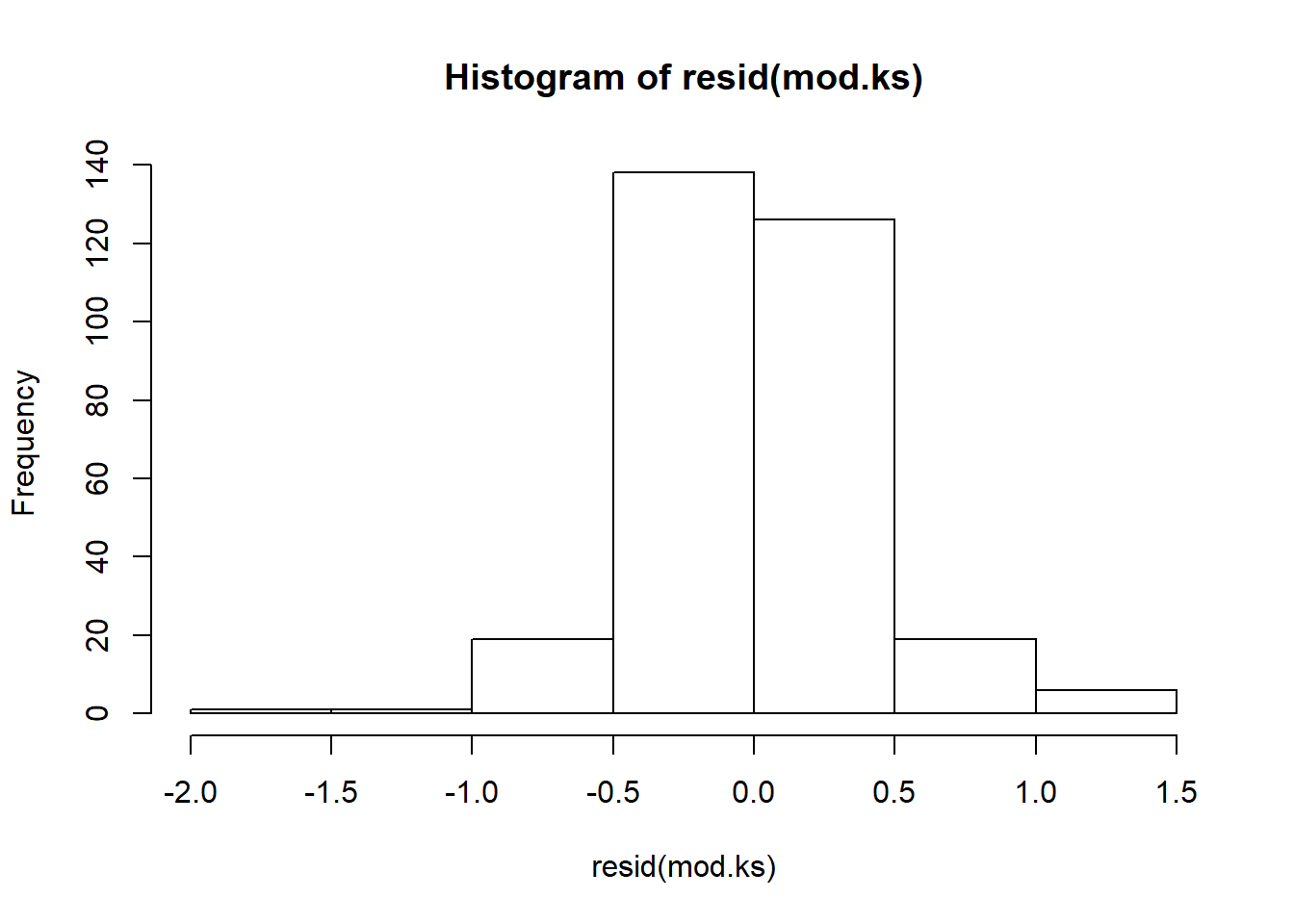``boxplot(resid(mod.ks), horizontal = TRUE)``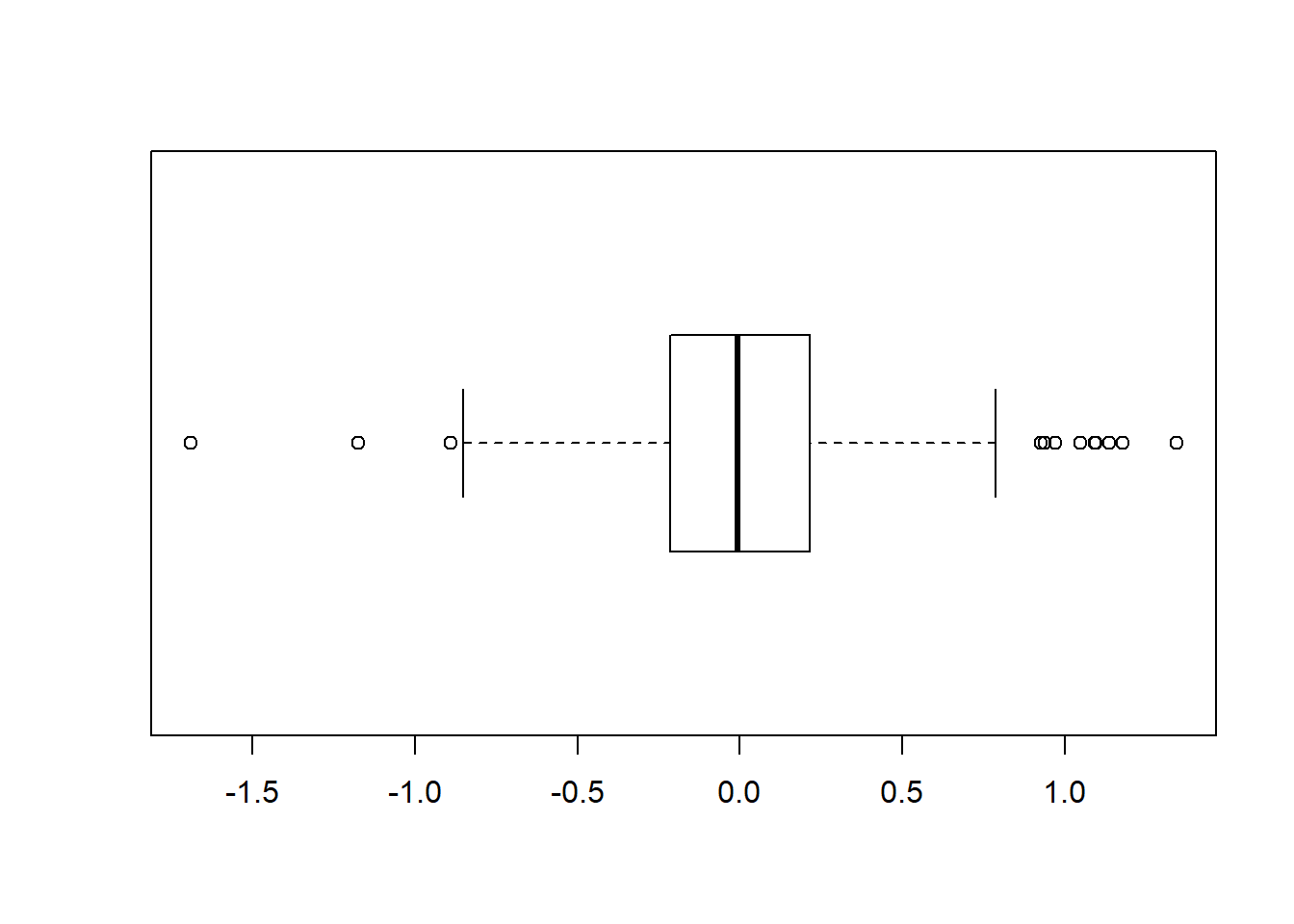``````qqnorm(resid(mod.ks))
qqline(resid(mod.ks))``````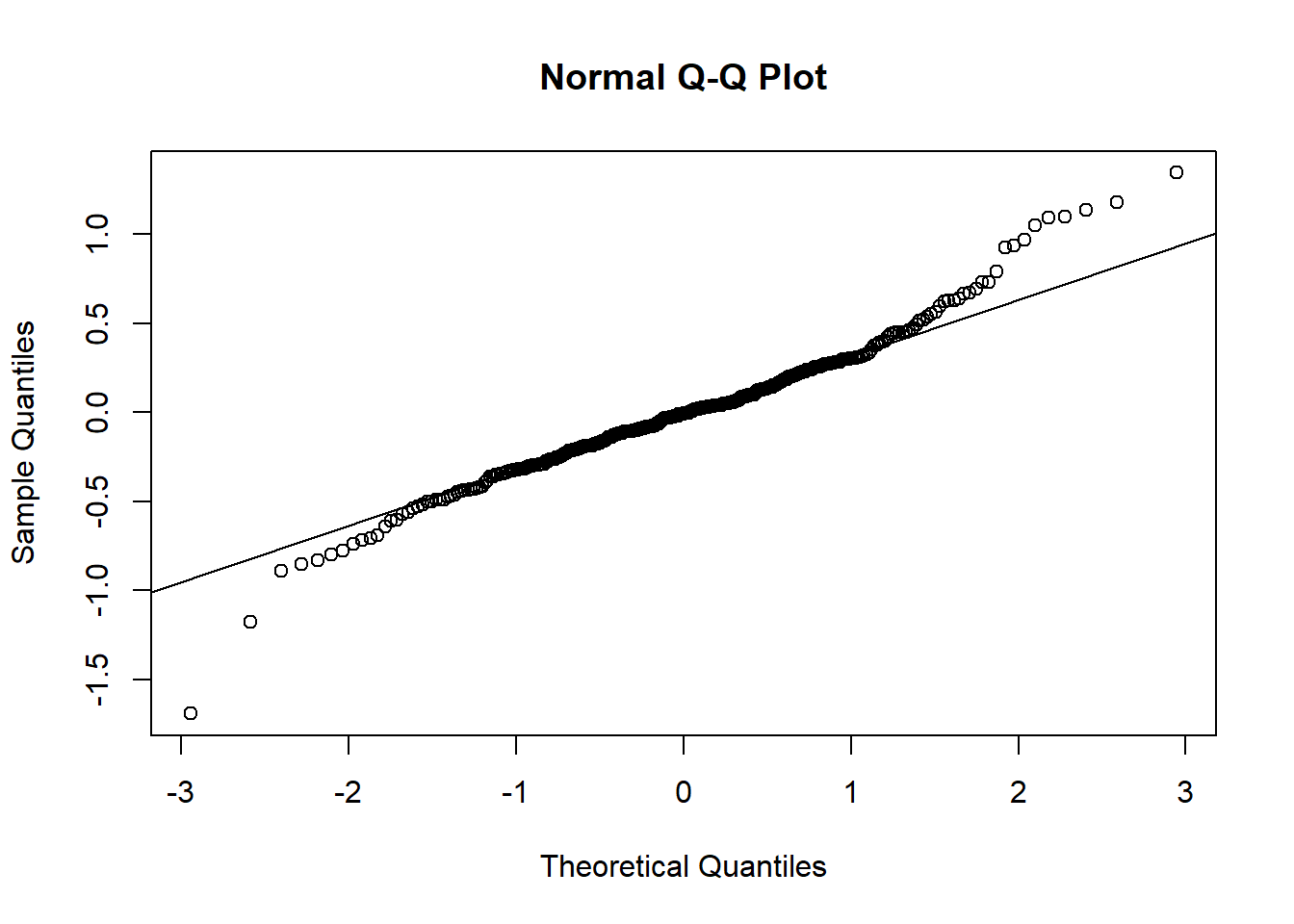This gives a somewhat different result. The sign of the `JurisCodeAB’ coefficient has flipped, implying that, all other things being equal, taxes are lower in Alberta. However, the dummy variable for province is only marginally significant once latitude and land size are added to the model. So Alberta property tax hawks can relax: differences between the two provinces seem to be driven by real causes and, if anything, they are getting a better deal than their counterparts in British Columbia.

##Stepwise refinement I use R’s stepwise refinement tool to generate a minimal model. It leaves the`JurisCodeAB` dummy in, so the refined model is the same as the kitchen sink model:

``mod.step <- step(mod.ks)``
``````## Start:  AIC=-591.69
## logTotalTax ~ Latitude + logPopulation + logTaxableLand + JurisCode
##
##                  Df Sum of Sq    RSS     AIC
## <none>                         44.51 -591.69
## - JurisCode       1      0.90  45.40 -587.49
## - Latitude        1      4.08  48.58 -566.52
## - logTaxableLand  1     54.26  98.77 -346.58
## - logPopulation   1    385.77 430.28  109.64``````
``summary(mod.step)``
``````##
## Call:
## lm(formula = logTotalTax ~ Latitude + logPopulation + logTaxableLand +
##     JurisCode, data = dat)
##
## Residuals:
##      Min       1Q   Median       3Q      Max
## -1.68944 -0.21529 -0.00729  0.21222  1.34324
##
## Coefficients:
##                 Estimate Std. Error t value Pr(>|t|)
## (Intercept)     3.409603   0.603968   5.645 3.77e-08 ***
## Latitude        0.058879   0.011140   5.286 2.39e-07 ***
## logPopulation   0.923108   0.017953  51.417  < 2e-16 ***
## logTaxableLand  0.176010   0.009128  19.283  < 2e-16 ***
## JurisCodeAB    -0.130879   0.052749  -2.481   0.0136 *
## ---
## Signif. codes:  0 '***' 0.001 '**' 0.01 '*' 0.05 '.' 0.1 ' ' 1
##
## Residual standard error: 0.382 on 305 degrees of freedom
## Multiple R-squared:  0.9346, Adjusted R-squared:  0.9338
## F-statistic:  1090 on 4 and 305 DF,  p-value: < 2.2e-16``````
``confint(mod.step)``
``````##                      2.5 %      97.5 %
## (Intercept)     2.22113061  4.59807532
## Latitude        0.03695901  0.08079939
## logPopulation   0.88777930  0.95843588
## logTaxableLand  0.15804931  0.19397146
## JurisCodeAB    -0.23467754 -0.02708111``````
``hist(resid(mod.step))``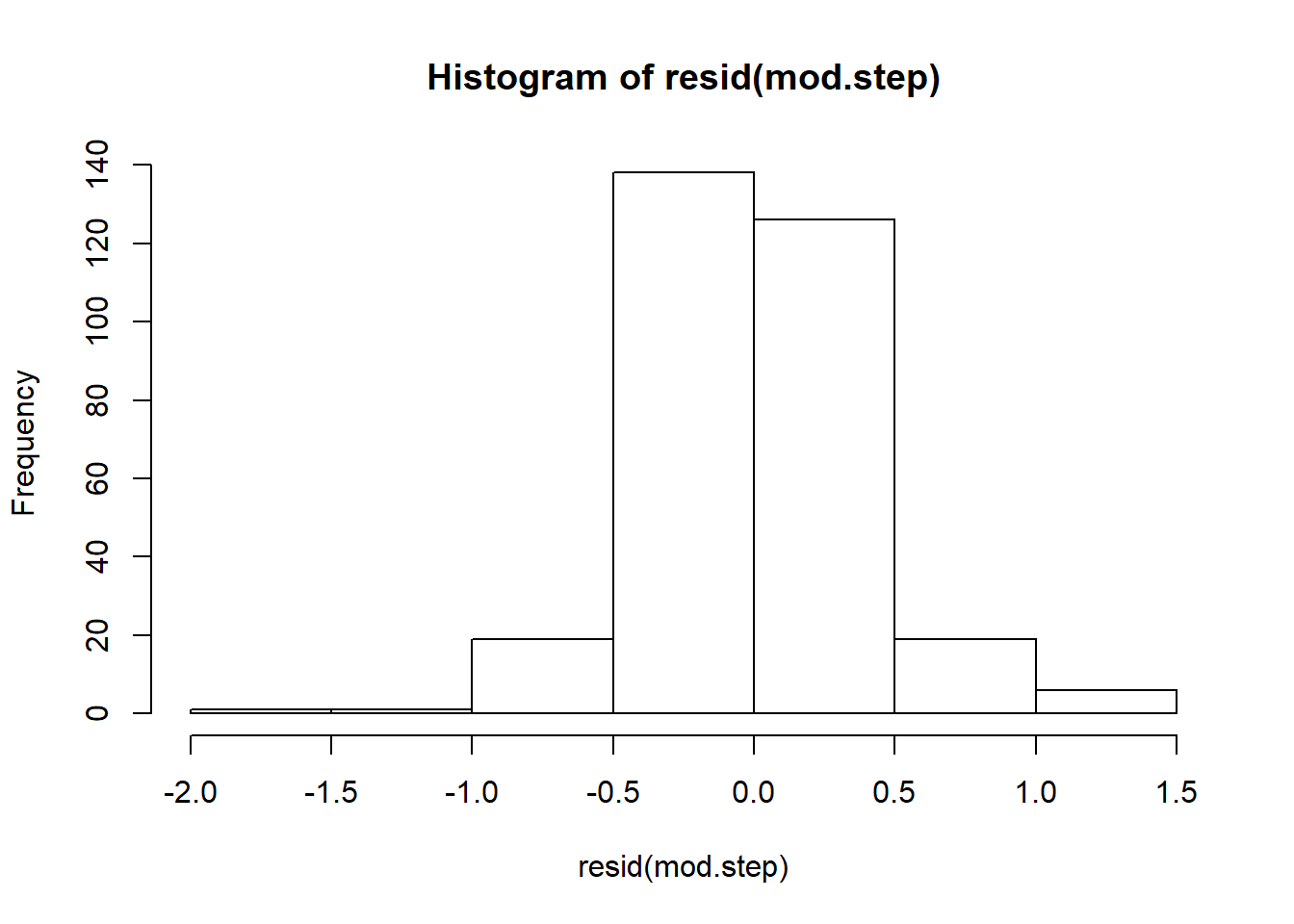``boxplot(resid(mod.step), horizontal = TRUE)````````qqnorm(resid(mod.step))
qqline(resid(mod.step))``````The \(R^2\) of this final model is a respectable 0.93. More critically, its fit plot looks convincing: the model does a pretty good job predicting overall taxation as a function of population, latitude, and physical extent.

``````plot(fitted(mod.step), dat\$logTotalTax, xlab="predicted value", ylab="observed value")
abline(0, 1)``````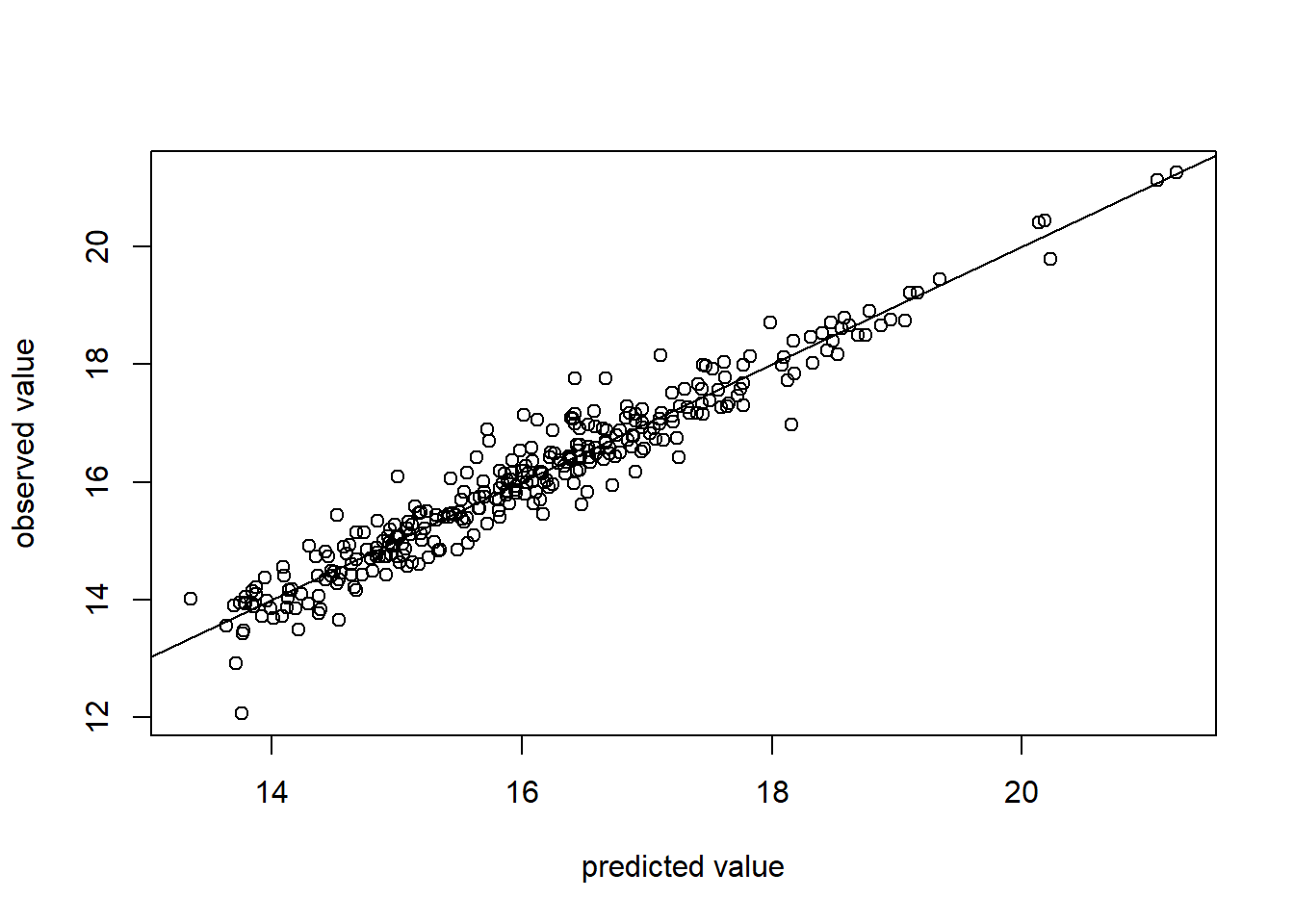## Ranking explanatory variables

I like to create a tornado diagram of the standardized coefficients. This gives a handy graphical guide to what is driving the explanatory model. In this case, we can see (not surprisingly) that population is the dominant explanatory variable:

``````mod.std <- lm.beta(mod.step)
tidy(mod.std) %>% ggplot() +
geom_col(mapping=aes(x=reorder(names, abs(x)), y=x), fill="blue") +
xlab("") +
ylab("Standardized Coefficient") +
coord_flip()``````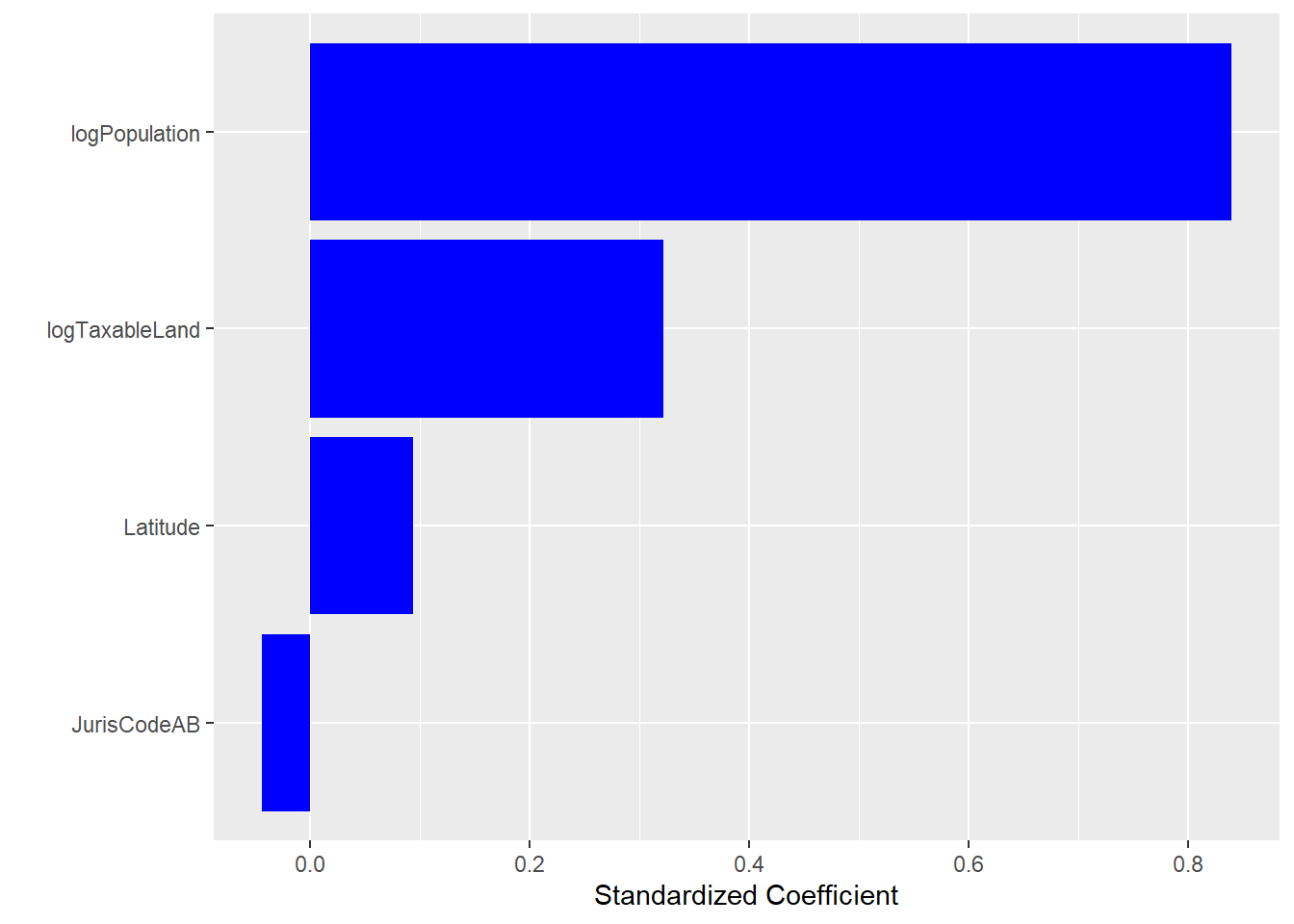#Evaluating municipalities Given this reasonably- accurate predictive model of taxation, we can invite controversy and abuse by comparing predicted taxation to observed taxation for each municipality. Conceptually, municipalities that fall below the 45\(^\circ\) fit line are taxing at a level lower than the model predicts whereas those above the fit line are taxing more than we might expect given their population, latitude, and physical size.

``````obs_max <- max(dat\$logTotalTax)
obs_min = min(dat\$logTotalTax)
mod.step %>% augment(data = dat) %>% ggplot(mapping = aes(x=.fitted, y=logTotalTax, color=JurisCode)) +
geom_point() +
ggtitle("Observed vs. Predicted Taxation") +
xlab("Predicted") +
ylab("Observed") +
xlim(obs_min, obs_max) +
ylim(obs_min, obs_max) +
geom_abline(slope=1, intercept=0, color="blue", size=1)``````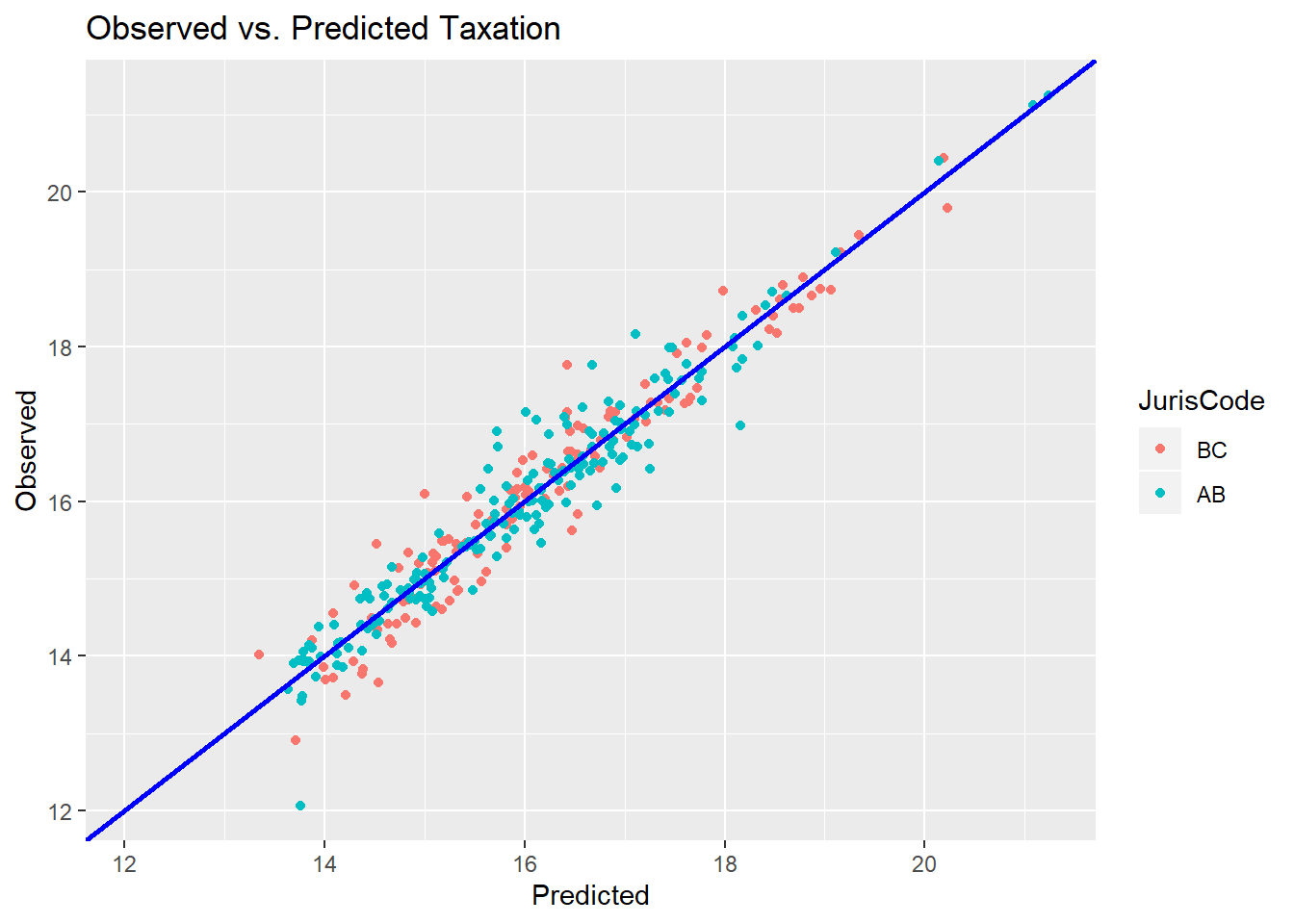I have not included municipality names on the fit plot because 310 overlapping labels makes the chart unreadable. An alternative is simply to list communities in order of the relative size of their deviation from the model. In the column chart below, I plot \({residual}/{fitted} * 100\) for each municipality. Thus, a community that taxed \$100K more than a predicted total taxation of \$1M would show on the plot as 10%. I do not show all 310 communities below but just a sample of 50. If you want the complete list, you can change the sample size in the R code or look at the follow-on Tableau visualizations in the blog post.

``````#Column (sampled)
mod.step %>% augment(data = dat) %>% sample_n(30, replace=FALSE) %>% mutate(predict_per = .resid/.fitted * 100) %>%
ggplot(mapping = aes(x=reorder(OrgNameFull, predict_per), y=predict_per, fill=JurisCode)) +
geom_col() +
xlab("") +
ylab("Relative Deviation from Model") +
coord_flip()``````